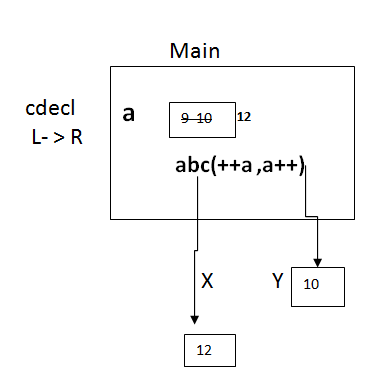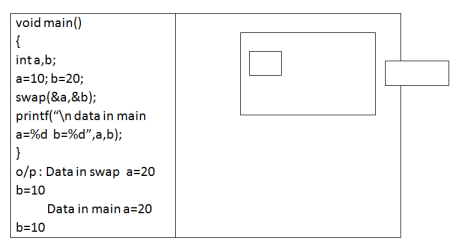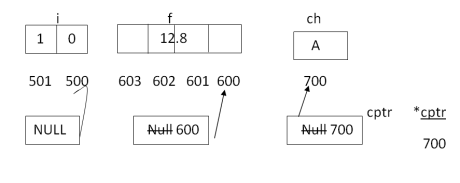Arguments in C
Ratings:
(4)
Views:0## Formal arguments & Actual arguments

In F’n declaratory (or) in f’n header, whatever the variables we are creating are called formal arguments or parameters.

• In f’n calling statement, whatever the data we are passing, those are called actual arguments or “Arguments” in C
• In order to call any f’n, if it is required specific num of parameters then we can’t invoke the f’n with less than or more than the required no. of arguments.

Note:

Where the implementation part of the f’n is available, it is called f’n definition.

• Always f’n definition contains body of the f’n
• In f’n definition, first line is called f’n declarator or f’n header.
• Where we are invoking the logic of the f’n , it is called f’n “ calling statement “

## Calling conversions:

• As a programmer, it is our responsibility to indicate the parameter creation sequence at the time of calling the f’n.
• Always calling conversions will decide in which sequence, parameters need to be created i.e left to right or right to left
• In ‘c’ programming language,

there are ‘2’ types of calling conversions are available e

• cdecl (- cdecl)
• pascal (- pascal)

### Cdecl calling conversion :

• When we are working with cdecl calling conversions, then parameters will be constructed from right to left.
• By default, any f’n calling conversion is “cdecl” only
• When we are working with “cdecl” calling conversion, then recommended placing the f’n name in lower case

## Pascal calling conversion :

• In pascal calling conversion, Arguments will pass towards from left to right
• Generally, pascal calling conversions are used in database programming.
• When we are working with pascal calling conversion, then recommended placing f’n name in upper case.
• Always formal arguments or parameters are initialized with actual arguments or arguments data at the time of calling the f’n.#### void cdecl abc(int x, int y)

``````{
printf(“n x=%d y=%d”, x,y);
}

void main()
{
int a;
a=10;
abc(++a,a++);
printf(“na=%d”,a);
}``````

o/p : x=12           y=10           a=12

#### void pascal xyz(int x, int y)

``````{
printf(“n x=%d     Y=%d”, x,y);
}

void main()
{
int a;
a=10;
xyz(a++,++a);
printf(“n%d”,a);
}

``````

o/p : x=10; y=12 a=12;

## Parameter passing techniques:

In ‘c’ programming language there are two types of parameters passing techniques are available i.e

• call by value or pass by value

### CALL by value :

• Whenever, we are calling a f’n by sending value type data, then it is called call by value.
• In call by value, actual arguments and formal arguments both are value type variable only.
• If any modifications occur on formal arguments in C, then those changes will not affect on actual arguments.

eg: printf(), pow(), sqrt(), cos(), delay(), text color()

• When we are calling a f’n by sending address type data , then it is called call by address.
• when we are working with call by address, then actual arguments are address, type, formal arguments are pointer type.
• If any modifications occur on formal arguments, then those changes will pass to actual arguments also.

eg:  scanf(), strcpy(),strrev(),strupr(),settime()

#### Void main()

``````{
int t;
t=1;
a=b;
b=t;
printf(“n a=%d    b=%d  ”,a,b);
}

void main()
{
int a,b;
a=10; b=20;
swap(a,b);
printf(“na=%d   b=%d”,a,b);
}``````

o/p : error  undefined symbol a,b

• According to storage classes of ‘c’ , a&b are auto variables which is constructed within the body of the main f’n, so we can’t access in swap f’n
• In order to call the swap f’n it doesn’t require any parameters but we are calling the f’n by using two arguments, so, it is not possible.

#### void swap (int a, int b)

``````{
int t;
t=a;
a=b;
b=t;
printf(“n data in swap a=%d   b=%d ”,a,b);
}``````

#### void main()

``````{
int a,b;
a=10, b=20;
swap(a,b);
printf(“n data in main a=%d  b=%d  ”,a,b);
}``````

o/p : data in swap  a=20       b-10 data in main  a=10       b=20

• in the above program, swap fin will work according to the call by value mechanism. That’s why no any modification of swap f’n will carry back to the main f’n
• In an implementation, when we are expecting the modification, then recommended creating the program by using the call by address mechanism.

#### void main(int*p, int*b)

``````{
int t;
t=*p1;        //t=1
*p1=*p2;   //a=b
*p2=t;        //b=t
printf(“n data in swap a=%d b=%d, *p1,*p2”);
}``````The above program swap f’n is working with the help of call by address mechanism, that why all the modification of the swap f’n will pass back to main f’n

Note : ‘c’ programming language does not supports call by reference.

• Call by reference is an oops feature that works with the help of a reference variable.
• ‘c’ language doesn’t have any reference variables, so call by reference is not possible.

Program to Call by reference

``````int power(int b,int e){int r=l,i;if(e<0)
return 0;
for(i=1;i<=e;i++)
r=r*b;
return r;
}
Void main()
{
Int a,b,p;
Clrscr();
Printf(“n Enter value of a:”);
Scanf(“%d”,&a);
Printf(“n Enter value of b:”)
Scanf(“%d”, &b);
//p=pow(a,b); <math.h>
P=power(a,b);
Printf(“n %d ^ %d value is : %d”,a,b,p);
Getch();
}``````

O/P : enter a value of a:2

Enter the value of b:5

25  value is : 32

• “returns” is a keyword, by using the return keyword, we can pass the control back to the calling place with arguments or without arguments
• In a f’n, we place any number of return statements, but at even any point of time, only one return statement executes.
• By using a return, statement, it is possible to return only one value by using f’n return type also, it is possible to return only one value.
• In an implementation, when we need to return more than one value from f’n , then recommended going for call by address
• By using call by address it is not possible to return multiple values but possible to collect multiple values.

#### int max(int x, int y)

``````{
if(x>y)
return x;
else
return Y;
}
Void main()
{
int m;
m=max(10,20);
printf(“n max value is %d”,m);
}``````

o/p : max value  is =20

#### int abc (int *p,int *p2)

``````{
int a=10,b=20,c=30;
a*=10+2;   //a=a*(10+2);
b%=6; //b=b%6;
c/3; //c=c/3;
*p1=b;                 //j=b;

*p2=c                   //k=c;
return a;
}
void main()
{
int i,j,k;
i=abc(&j,&k);
printf(“ni=%d    j=%d           k=%d”,i,j.k);
getch();
}``````

o/p : i=20; j=2;   k=10 Enter  a value =5 5 fact value is =120#### void main()

``````{
int n,f;
// int fact(int); declaration
clrscr();
printf(“Enter a value”);
scanf(“%d”,&n);
f=fct(n);
printf(“n %d fact value is : %d”,n,f);
getch();
}
int fact (int n)
{
int r=1,i;
for(“i=n,i>=1;i--”);
r=r*i;
return r;``````

According to K and RC standard above prog is valid because whenever the f’n return type is an integer parameter type is other than float then doesn’t require to go for forwarding declaration.

According to ANSI-C standard, the above program is an error because the forward declaration of a f’n must be required whenever we are defining a f’n after calling.

### For an in-depth understanding of Pointers click on